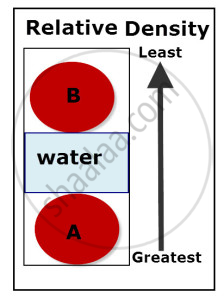Share

# Relative Density

#### notes

Relative density:The relative density of a substance is the ratio of its density to that of water.

Relative density is a ratio so it has no unit.

"Relative density" = "density of substance" / "density of water"

The specific gravity of a substance will let us know if it will float or sink, it gives us the idea about relative mass or relative density. If the specific gravity of a substance is below 1 then it will float and if it is greater than 1 it will sink.

S